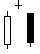# AbandonedBaby

 Description An Abandoned Baby pattern is a Morning or Evening Star in which the star, or center candlestick, gaps away from both the preceding and following candlesticks. Such a star is considered major reversal pattern. The Abandoned Baby pattern is extremely rare. Formula AbandonedBaby(INPUT,Stringency=5,LRRange=10,LRType=3,SmallForm=10)= begin   retval = NONUM   targetBar = 0   pnt0 = targetBar   pnt1 = targetBar + 1   pnt2 = targetBar + 2   ppClose = GetPrice(\$1, BATE_CLOSE, pnt2, 0)   ppHigh = GetPrice(\$1, BATE_HIGH, pnt2, 0)   ppLow = GetPrice(\$1, BATE_LOW, pnt2, 0)   pHigh = GetPrice(\$1, BATE_HIGH, pnt1, 0)   pOpen = GetPrice(\$1, BATE_OPEN, pnt1, 0)   pClose = GetPrice(\$1, BATE_CLOSE, pnt1, 0)   pLow = GetPrice(\$1, BATE_LOW, pnt1, 0)   cOpen = GetPrice(\$1, BATE_OPEN, targetBar, 0)   cHigh = GetPrice(\$1, BATE_HIGH, targetBar, 0)   cLow = GetPrice(\$1, BATE_LOW, targetBar, 0)     if BlackBody(\$1, pnt0) == TRUE then begin     if WhiteBody(\$1, pnt2) == TRUE then begin       if pLow > ppHigh then begin         if cHigh < pLow then begin           if SmallRealBody(\$1, pnt1, SmallForm) then begin             if Stringency >= 5 then retval = '5'|clr_red|above|ftiny|arrow|vertical             if DojiForm(\$1, pnt1) then begin               if Stringency >= 4 then retval = '4'|clr_red|above|ftiny|arrow|vertical               if cOpen < pLow then begin                 if Stringency >= 3 then retval = '3'|clr_red|above|ftiny|arrow|vertical                 if LongWhiteForm(\$1, pnt2) then begin                   if Stringency >= 2 then retval = '2'|clr_red|above|ftiny|arrow|vertical                   if LongBlackForm(\$1, pnt1) then begin                     retval = 'Black Abandoned Baby'|clr_black|above|ftiny|arrow|vertical                   end                 end               end             end           end         end       end     end   end     if WhiteBody(\$1, pnt0) == TRUE then begin     if BlackBody(\$1, pnt2) == TRUE then begin       if pHigh < ppLow then begin         if cLow > pHigh then begin           if SmallRealBody(\$1, pnt1, SmallForm) then begin             if Stringency >= 5 then retval = '5'|clr_green|below|ftiny|arrow|vertical             if cOpen > pHigh then begin               if Stringency >= 4 then retval = '4'|clr_green|below|ftiny|arrow|vertical               if LongBlackForm(\$1, pnt2) OR DojiForm(\$1, pnt1) OR LongWhiteForm(\$1, pnt1) then begin                 if Stringency >= 3 then retval = '3'|clr_green|below|ftiny|arrow|vertical                 if LongWhiteForm(\$1, pnt1) then begin                   if Stringency >= 2 then retval = '2'|clr_green|below|ftiny|arrow|vertical                   if Bearish(\$1, pnt2, LRRange, LRType) == TRUE then begin                     retval = 'White Abandoned Baby'|clr_black|below|ftiny|arrow|vertical                   end                 end               end             end           end         end       end     end   end   retval end Parameters INPUT The INPUT directive makes this formula available as a Formula Overlay. INPUT refers to the instrument in the chart. INPUT does not become an overlay parameter.   Stringency The Stringency argument is used by nearly all candlestick overlays. It controls how many tests must pass in the evaluation of an Abandoned Baby. The lower the number, the more strict the interpretation.   The Stringency argument is an overlay parameter. You can adjust it using the Parameters dialog. The default is 5.   LRRange The AbandonedBaby overlay uses the LR() function with either a continuous or quadratic calculation to determine how the market is trending. The LRRange (Linear Regression Range) parameter is the number of points in the Linear Regression calculation. The default is 10.   LRType The LRType parameter has two possible settings: 2 and 3, or continuous linear regression and quadratic linear regression, respectively. The default is 3, or quadratic.   SmallForm The SmallForm parameter represents a percentage. This parameter serves as the basis for determining whether a candlestick is Doji or near Doji. The smaller the value assigned to SmallForm the more stringent the test. The default is 10, or ten percent. Return Value The return value is a string plot and varies depending on the value of the Stringency parameter.  The lower the number, the stronger the pattern.   Bearish indicators are rendered in red above the candlestick. Bullish indicators are rendered in green below the candlestick.   The string plot for a fully qualified Abandoned Baby is "White Abandoned Baby" or "Black Abandoned Baby," depending on whether the Abandoned Baby is bullish or bearish, respectively. ExamplesComments NA